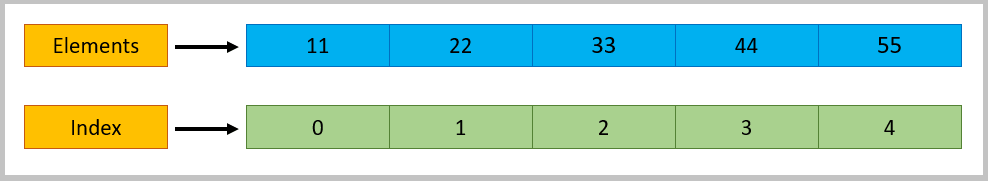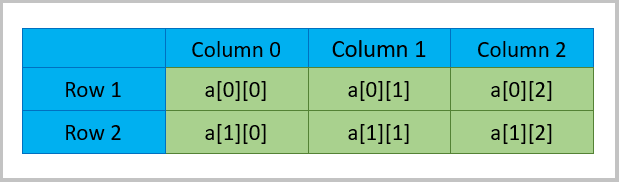# Arrays in Java

Collection of similar type of elements is known as Array. Array in Java is an Object that holds fixed number of values of a similar data types which means an array of int will contain only integers, an array of string will contain only strings etc.. The length of an array is established when the array is created. After creation, its length is fixed. Array is a index based and its index starts from 0 which means the first element of an array is stored at 0 index. Array holds primitive types as well as object references.

Syntax:

or

Example:

also

‘arr’ is a collection of 5 integers

int[] arr represents that ‘arr’ is an array of type integer
[] represents an array
new int represents it as array of 5 integers

As per the above example, we have 5 elements in the array such as 11, 22, 33, 44, 55.

Arrays index starts from 0 so the first element (11) of this array has index 0, second element (22) has index 1, … last element (55 which is fifth element) has index of 4.Which means,

arr(0) = 11;
arr(1) = 22;
arr(2) = 33;
arr(3) = 44;
arr(4) = 55;

If we know the elements of an array, then we can also declare the array and assign values to the its variables as:

Array Example 1:

Output:

Array Example 2:

Output:

Finding Length of Array:

Output:

Array with For Loop:

Output:

Array with For Each Loop:

Here, the variable a will go to every element of the array arr and will take its value.
So, in the first iteration, a is the 1st element of the array arr i.e. 11.
In the second iteration, it is the 2nd element i.e. 22 and so on.

Output:

2D Array:

2D Arrays are same like 1D Array but it consists of rows and columns.

2D Array Declaration:

Syntax:

Example:

Here, arr is a 2-D array of type int which consists of 2 rows and 3 columns.

Lets see how the rows and columns work2D Array Initialization:

Declaring 2D array and then assigning values to its elements

Output:

Declaring and assigning values at the same time

Here, the value of a is 11, a is 22, a is 33, a is 44, a is 55 and a is 66.

Output:

Limitations of Array:

1. Arrays wont allow multiple data type.
2. In Array, we can store only fixed size of elements.

To overcome this problem, we can use ArrayList, List, Set, Collections. We will see those in next sections.Rajkumar SM is a founder of SoftwareTestingMaterial. He is a certified Software Test Engineer by profession and blogger & youtuber by choice. He has an extensive experience in the field of Software Testing. He writes here about Software Testing which includes both Manual and Automation Testing. He loves to be with his wife and cute little kid 'Freedom'.